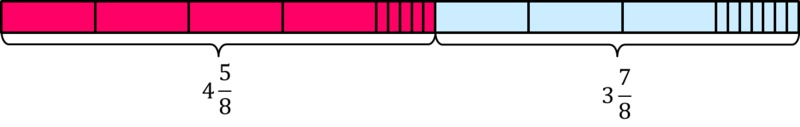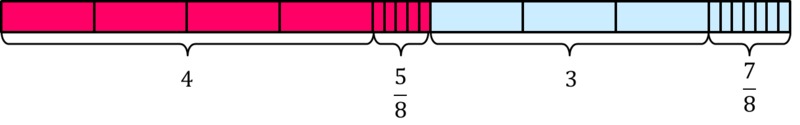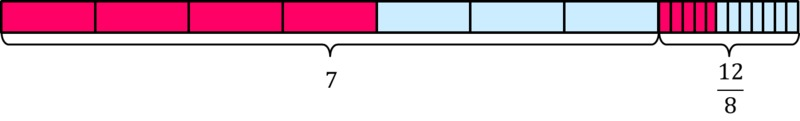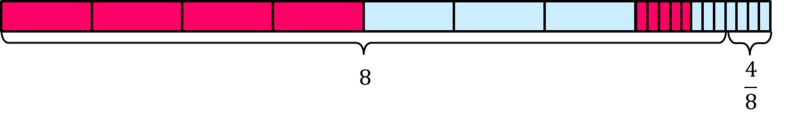# Cynthia's Perfect Punch

Alignments to Content Standards: 4.NF.B.3.c

Cynthia is making her famous "Perfect Punch" for a party. After looking through the recipe, Cynthia knows that she needs to mix $4\frac58$ gallons of fruit juice concentrate with $3\frac78$ gallons of sparkling water.

1. Just as she is about to get started she realizes that she only has one 10-gallon container to use for mixing. Will this container be big enough to hold all the ingredients?
2. How much punch will this recipe make?

## IM Commentary

The purpose of this task is for students to estimate and compute sums of mixed numbers in a context. A common error for students who are not used to representing fractions with diagrams or on the number line is to add the numerators and the denominators of fractions together like so:

$$\frac58+\frac78 = \frac{5+7}{8+8}$$

Students still struggling with this should draw diagrams or number lines to represent the sums--graph paper is very useful for this as students can quickly and accurately represent the fractions in this task.

Note that the equivalence between $\frac48$ and $\frac12$ is a third-grade expectation. If students are still struggling with that, please see the tasks that illustrate standard 3.NF.3.

## Solution

1. The container is large enough to hold all of the ingredients. Perhaps the easiest way to see this is by observing that $4\frac58$ is less than 5 and $3\frac78$ is less than 4, so $4\frac58 + 3\frac78$ is less than 9. Since there are less than 9 gallons of ingredients altogether they will certainly all fit in a 10-gallon container.

2. To see how much total punch is made we need to add the amount of lemon lime soda to the amount of fruit juice. The picture below represents $4\frac58 + 3\frac78$.We can write the mixed numbers as a sum of a whole number and a fraction.

$$4\frac58 + 3\frac78 = (4 + \frac{5}{8}) + (3 + \frac{7}{8})$$Since addition is commutative and associative, we can add the numbers in any order we wish. Let's add the whole numbers together and the fractions together.

$$4+\frac58+3+\frac78 = 4+3+\frac58+\frac78$$$$4+3+\frac58+\frac78 = 7 + \frac{5+7}{8} = 7 + \frac{12}{8}$$

Next we can re-write $\frac{12}{8}$ as a mixed number...

$$7 + \frac{12}{8} = 7 + \frac{8+4}{8} = 7 + \frac88 + \frac48 = 7+1+\frac48$$

and add the whole numbers once again.$$7+1+\frac48 = 8+\frac48$$

Since $\frac48=\frac12$, we can write the sum as $8\frac12$. So we see that this recipe makes $8\frac12$ gallons of punch.